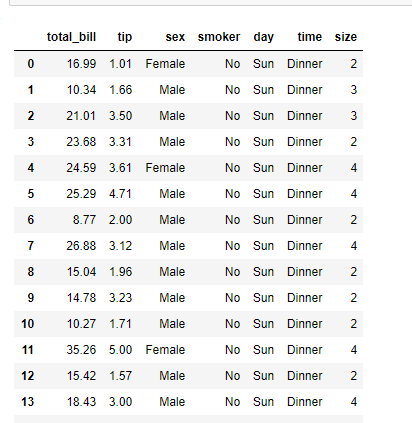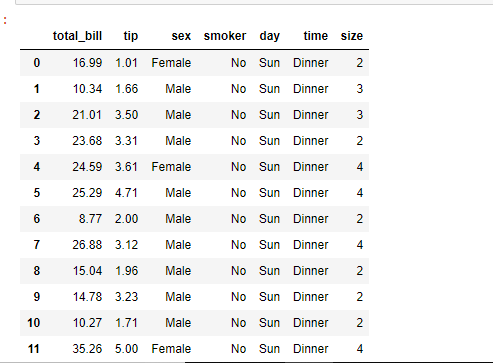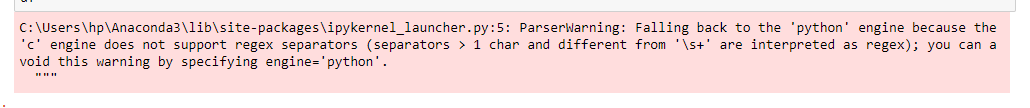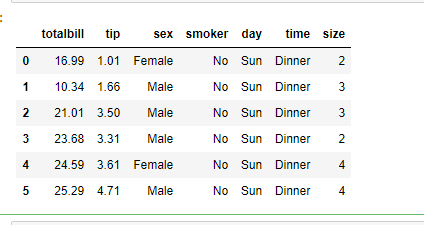# How to read a CSV file to a Dataframe with custom delimiter in Pandas?

Python is a good language for doing data analysis because of the amazing ecosystem of data-centric python packages. pandas package is one of them and makes importing and analyzing data so much easier.

Here, we will discuss how to load a csv file into a Dataframe. It is done using a pandas.read_csv() method. We have to import pandas library to use this method.

Syntax: pd.read_csv(filepath_or_buffer, sep=’, ‘, delimiter=None, header=’infer’, names=None, index_col=None, usecols=None, squeeze=False, prefix=None, mangle_dupe_cols=True, dtype=None, engine=None, converters=None, true_values=None, false_values=None, skipinitialspace=False, skiprows=None, nrows=None, na_values=None, keep_default_na=True, na_filter=True, verbose=False, skip_blank_lines=True, parse_dates=False, infer_datetime_format=False, keep_date_col=False, date_parser=None, dayfirst=False, iterator=False, chunksize=None, compression=’infer’, thousands=None, decimal=b’.’, lineterminator=None, quotechar='”‘, quoting=0, escapechar=None, comment=None, encoding=None, dialect=None, tupleize_cols=None, error_bad_lines=True, warn_bad_lines=True, skipfooter=0, doublequote=True, delim_whitespace=False, low_memory=True, memory_map=False, float_precision=None)

Some Useful parameters are given below :

Parameter Use
filepath_or_buffer URL or Dir location of file
sep Stands for seperator, default is ‘, ‘ as in csv(comma seperated values)
index_col This parameter is use to make passed column as index instead of 0, 1, 2, 3…r
header This parameter is use to make passed row/s[int/int list] as header
use_cols This parameter is Only uses the passed col[string list] to make data frame
squeeze If True and only one column is passed then returns pandas series
skiprows This parameter is use to skip passed rows in new data frame
skipfooter This parameter is use to skip Number of lines at bottom of file

This method uses comma ‘, ‘ as a default delimiter but we can also use a custom delimiter or a regular expression as a separator.

Example 1 : Using the `read_csv()` method with default separator i.e. comma(, )

 `# Importing pandas library ` `import` `pandas as pd ` ` `  `# Using the function to load  ` `# the data of example.csv  ` `# into a Dataframe df ` `df ``=` `pd.read_csv(``'example1.csv'``) ` ` `  `# Print the Dataframe ` `df `

Output:Example 2: Using the `read_csv()` method with ‘_’ as a custom delimiter.

 `# Importing pandas library ` `import` `pandas as pd ` ` `  `# Load the data of example.csv  ` `# with '_' as custom delimiter ` `# into a Dataframe df ` `df ``=` `pd.read_csv(``'example2.csv'``,  ` `                   ``sep ``=` `'_'``,  ` `                   ``engine ``=` `'python'``) ` ` `  `# Print the Dataframe ` `df `

Output:Note:While giving a custom specifier we must specify engine=’python’ otherwise we may get a warning like the one given below:Example 3 : Using the `read_csv()` method with tab as a custom delimiter.

 `# Importing pandas library ` `import` `pandas as pd ` ` `  `# Load the data of example.csv ` `# with tab as custom delimiter ` `# into a Dataframe df ` `df ``=` `pd.read_csv(``'example3.csv'``,  ` `                   ``sep ``=` `'\t'``,  ` `                   ``engine ``=` `'python'``) ` ` `  `# Print the Dataframe ` `df `

Output:Example 4 : Using the `read_csv()` method with regular expression as custom delimiter.

Let’s suppose we have a csv file with multiple type of delimiters such as given below.

totalbill_tip, sex:smoker, day_time, size
16.99, 1.01:Female|No, Sun, Dinner, 2
10.34, 1.66, Male, No|Sun:Dinner, 3
21.01:3.5_Male, No:Sun, Dinner, 3
23.68, 3.31, Male|No, Sun_Dinner, 2
24.59:3.61, Female_No, Sun, Dinner, 4
25.29, 4.71|Male, No:Sun, Dinner, 4

To load such file into a dataframe we use regular expression as a separator.

 `# Importing pandas library ` `import` `pandas as pd ` ` `  `# Load the data of example.csv ` `# with regular expression as  ` `# custom delimiter into a  ` `# Dataframe df ` `df ``=` `pd.read_csv(``'example4.csv'``,  ` `                   ``sep ``=` `'[:, |_]'``,  ` `                   ``engine ``=` `'python'``) ` ` `  `# Print the Dataframe ` `df `

Output:Attention geek! Strengthen your foundations with the Python Programming Foundation Course and learn the basics.

To begin with, your interview preparations Enhance your Data Structures concepts with the Python DS Course.

My Personal Notes arrow_drop_upCheck out this Author's contributed articles.

If you like GeeksforGeeks and would like to contribute, you can also write an article using contribute.geeksforgeeks.org or mail your article to contribute@geeksforgeeks.org. See your article appearing on the GeeksforGeeks main page and help other Geeks.

Please Improve this article if you find anything incorrect by clicking on the "Improve Article" button below.

Article Tags :

Be the First to upvote.

Please write to us at contribute@geeksforgeeks.org to report any issue with the above content.